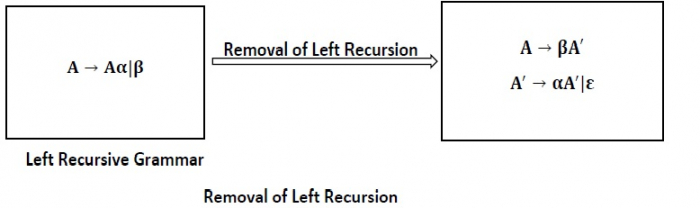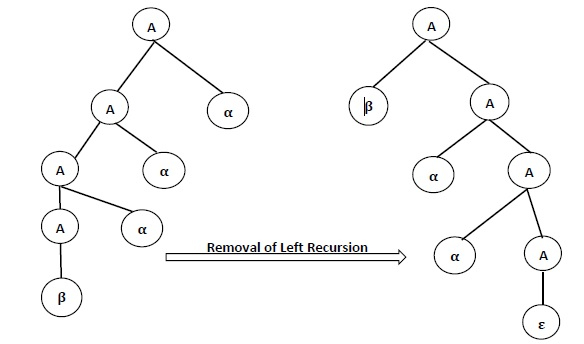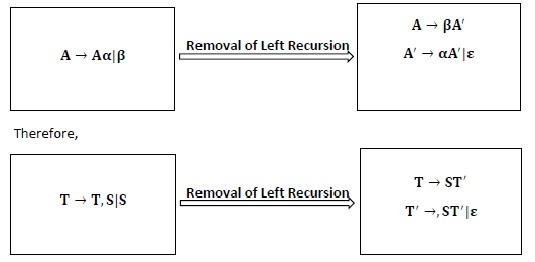# What is Left Recursion and how it is eliminated?

Compiler DesignProgramming LanguagesComputer Programming

#### Web Design for Beginners: Build Websites in HTML & CSS 2022

68 Lectures 8 hours

#### HTML5 & CSS3 Site Design

61 Lectures 6 hours

A Grammar G (V, T, P, S) is left recursive if it has a production in the form.

A → A α |β.

The above Grammar is left recursive because the left of production is occurring at a first position on the right side of production. It can eliminate left recursion by replacing a pair of production with

A → βA′

A → αA′|ϵ

Elimination of Left Recursion

Left Recursion can be eliminated by introducing new non-terminal A such that.This type of recursion is also called Immediate Left Recursion.

In Left Recursive Grammar, expansion of A will generate Aα, Aαα, Aααα at each step, causing it to enter into an infinite loopThe general form for left recursion is

A → Aα1|Aα2| … . |Aαm12| … . . βn

can be replaced by

A → β1A′|β2A′| … . . | … . . |βnA′

A → α1A′|α2A′| … . . |αmA′|ε

Example1 − Consider the Left Recursion from the Grammar.

E → E + T|T

T → T * F|F

F → (E)|id

Eliminate immediate left recursion from the Grammar.

Solution

Comparing E → E + T|T with A → A α |β

 E → E +T | T A → A α | Β

∴ A = E, α = +T, β = T

∴ A → A α |β is changed to A → βA′and A′ → α A′|ε

∴ A → βA′ means E → TE′

A′ → α A′|ε means E′ → +TE′|ε

Comparing T → T ∗ F|F with A → Aα|β

 T → T *F | F A → A α | β

∴ A = T, α =∗ F, β = F

∴ A → β A′ means T → FT′

A → α A′|ε means T′ →* FT′|ε

Production F → (E)|id does not have any left recursion

∴ Combining productions 1, 2, 3, 4, 5, we get

E → TE′
E′ → +TE′| ε
T → FT′
T →* FT′|ε
F → (E)| id

Example2 − Eliminate the left recursion for the following Grammar.

S → a|^|(T)

T → T, S|S

Solution

We have immediate left recursion in T-productions.

Comparing T → T, S|S With A → A α | β where A = T, α =, S and β = S∴ Complete Grammar will be


S→ a|^(T)
T→ ST′
T′ →,ST′| ε


Example3 − Eliminate the left recursion from the grammar

E → E + T|T

T → T * F|F

F → (E)|id

Solution

The production after removing the left recursion will be

E → TE′

E′ → +TE′| ∈

T → FT′

T′ →∗ FT′| ∈

F → (E)|id

Example4 − Remove the left recursion from the grammar

E → E(T)|T

T → T(F)|F

F → id

Solution

Eliminating immediate left-recursion among all Aα productions, we obtain

E → TE′

E → (T)E′|ε

T → FT′

T′ → (F)T′|ε

F → id